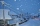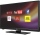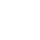# Fraction calculator

The calculator performs basic and advanced operations with fractions, expressions with fractions combined with integers, decimals, and mixed numbers. It also shows detailed step-by-step information about the fraction calculation procedure. Solve problems with two, three, or more fractions and numbers in one expression.

## Result:

### 73/8 + 4 = 91/8 = 11 3/8 = 11.375

Spelled result in words is ninety-one eighths (or eleven and three eighths).

### How do you solve fractions step by step?

1. Conversion a mixed number 7 3/8 to a improper fraction: 7 3/8 = 7 3/8 = 7 · 8 + 3/8 = 56 + 3/8 = 59/8

To find new numerator:
a) Multiply the whole number 7 by the denominator 8. Whole number 7 equally 7 * 8/8 = 56/8
b) Add the answer from previous step 56 to the numerator 3. New numerator is 56 + 3 = 59
c) Write a previous answer (new numerator 59) over the denominator 8.

Seven and three eighths is fifty-nine eighths
2. Add: 59/8 + 4 = 59/8 + 4/1 = 59/8 + 4 · 8/1 · 8 = 59/8 + 32/8 = 59 + 32/8 = 91/8
For adding, subtracting, and comparing fractions, it is suitable to adjust both fractions to a common (equal, identical) denominator. The common denominator you can calculate as the least common multiple of both denominators - LCM(8, 1) = 8. In practice, it is enough to find the common denominator (not necessarily the lowest) by multiplying the denominators: 8 × 1 = 8. In the next intermediate step, the fraction result cannot be further simplified by canceling.
In words - fifty-nine eighths plus four = ninety-one eighths.

#### Rules for expressions with fractions:

Fractions - use the slash “/” between the numerator and denominator, i.e., for five-hundredths, enter 5/100. If you are using mixed numbers, be sure to leave a single space between the whole and fraction part.
The slash separates the numerator (number above a fraction line) and denominator (number below).

Mixed numerals (mixed fractions or mixed numbers) write as non-zero integer separated by one space and fraction i.e., 1 2/3 (having the same sign). An example of a negative mixed fraction: -5 1/2.
Because slash is both signs for fraction line and division, we recommended use colon (:) as the operator of division fractions i.e., 1/2 : 3.

Decimals (decimal numbers) enter with a decimal point . and they are automatically converted to fractions - i.e. 1.45.

The colon : and slash / is the symbol of division. Can be used to divide mixed numbers 1 2/3 : 4 3/8 or can be used for write complex fractions i.e. 1/2 : 1/3.
An asterisk * or × is the symbol for multiplication.
Plus + is addition, minus sign - is subtraction and ()[] is mathematical parentheses.
The exponentiation/power symbol is ^ - for example: (7/8-4/5)^2 = (7/8-4/5)2

#### Examples:

subtracting fractions: 2/3 - 1/2
multiplying fractions: 7/8 * 3/9
dividing Fractions: 1/2 : 3/4
exponentiation of fraction: 3/5^3
fractional exponents: 16 ^ 1/2
adding fractions and mixed numbers: 8/5 + 6 2/7
dividing integer and fraction: 5 ÷ 1/2
complex fractions: 5/8 : 2 2/3
decimal to fraction: 0.625
Fraction to Decimal: 1/4
Fraction to Percent: 1/8 %
comparing fractions: 1/4 2/3
multiplying a fraction by a whole number: 6 * 3/4
square root of a fraction: sqrt(1/16)
reducing or simplifying the fraction (simplification) - dividing the numerator and denominator of a fraction by the same non-zero number - equivalent fraction: 4/22
expression with brackets: 1/3 * (1/2 - 3 3/8)
compound fraction: 3/4 of 5/7
fractions multiple: 2/3 of 3/5
divide to find the quotient: 3/5 ÷ 2/3

The calculator follows well-known rules for order of operations. The most common mnemonics for remembering this order of operations are:
PEMDAS - Parentheses, Exponents, Multiplication, Division, Addition, Subtraction.
BEDMAS - Brackets, Exponents, Division, Multiplication, Addition, Subtraction
BODMAS - Brackets, Of or Order, Division, Multiplication, Addition, Subtraction.
GEMDAS - Grouping Symbols - brackets (){}, Exponents, Multiplication, Division, Addition, Subtraction.
Be careful, always do multiplication and division before addition and subtraction. Some operators (+ and -) and (* and /) has the same priority and then must evaluate from left to right.

## Fractions in word problems:7 is added to the sum of 4/5 and 6/7
• SamuelSamuel has 1/3 of a bag of rice and Isabella has a 1/2 bag of rice. What fraction of are bag of rice do they have altogether?
• Sum three fractionsWork out the sum of 1/4, 1/5 and 3/10.What is the sum of 2/3 and 3/10?
• How many 3How many hours the Andersons watched TV in all Wednesday 3/1 hr Thursdays 2/3 hr Friday 4/5 hr Saturday 3/4 hr
• Sum of 18Sum of two fractions is 4 3/7. If one of the fractions is 2 1/5 find the other one .2 and 1/8th plus 1 and 1/3rd =Jade was baking cupcakes for her class. She has 4 5/4 cups of flour.  For one batch, she used 1 2/3 cups of flour.  On another batch, she used 7/9 of a cup of flour.  How much flour does Jade have left after making the two batches of cupcakes?Liza, a store owner, buys 560 notebooks. He sold 3/8 of the notebook, and then she adds the stock of a notebook of 1/4 of the number of notebooks she bought. What is the total number of notebooks she purchased?Alice creates a circular vegetable garden. Tomatoes are planted in 1/3 of the circular garden, carrots are planted in 2/5 of the circular garden, and green peppers are planted in 1/10 of the circular garden. What fraction represents the remaining unplanteA dump truck bought 1/3 of a ton of rock on the first trip, 1/2 of a ton on the second trip, and 4/5 of a ton on the third trip. What was the total weight of the rock?3 3/4 + 2 3/5 + 5 1/2 Show your solution.What is the sum of 2/3+3/5?